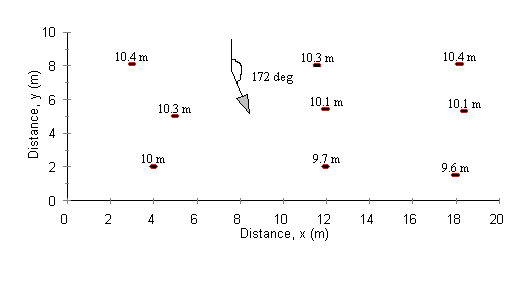Groundwater Flow Direction and Gradient Calculator

Enter heads at x, y locations. Compute groundwater flow direction, gradient, and rate. Confined or unconfined aquifer.

Register to enable "Calculate" button for groundwater gradient and flow.

Units in groundwater gradient calculator: cfs=ft3/s, cm=centimeter, ft=foot, gal=U.S.gallon, gpd=U.S. gallon per day, gpm=U.S. gallon per minute, km=kilometer, m=meter, s=second.

Plan View of Example Head Measurements in a Confined Aquifer. Results of calculation:  s = 172 degrees.  dh/ds = -0.0971 m/m.Groundwater Gradient and Groundwater Flow Direction Introduction
The groundwater flow gradient and direction calculator allows the user to enter measured well (piezometer) levels in up to 10 groundwater observation wells. The results are most reliable if all of the groundwater wells are screened in the same formation and there are no discontinuities in the subsurface in the vicinity of the wells. For a confined aquifer, a geometric plane is fit through the (x, y, head) data. Then, the maximum downward slope of the plane is computed and reported as the groundwater gradient, dh/ds. The direction of the maximum slope of the plane is computed and reported as the groundwater flow direction, s. Groundwater flow direction is reported as degrees clockwise from the positive y-axis defined by your x,y locations. For an unconfined aquifer, the calculation squares the input heads, then fits a plane through these squared heads. The maximum groundwater gradient and flow direction are based on the plane formed by the squared heads. Since dh/ds varies with position in an unconfined aquifer, we report h(dh/ds), which is constant. Darcy's law is then used to compute groundwater flow per unit width of the formation.

The equation for a plane is z = A x + B y + C, where A, B, and C are constants obtained by performing a best least squares fit of the plane equation through the input data. For a confined aquifer z = h and for an unconfined aquifer z = h2. To determine flow per unit width of aquifer, Darcy's law is used:
For a confined aquifer, Q = - K H dh/ds
For an unconfined aquifer, Q = - K h dh/ds
The reason we use z = h2 for an unconfined aquifer is because Q = - K h dh/ds = -0.5 K d(h2)/ds, so a plane in h2 exists.

Groundwater Flow and Gradient Variable Definitions
The variables used on this web page are shown below. [L]=Length units and [T]=Time units.
A, B, C = constants determined by fitting the plane equation,   z = A x + B y + C,  to the input data.
dh/ds = Steepest groundwater hydraulic gradient along plane formed by input data for a confined aquifer [L/L]. Value will be negative since groundwater flows from high head to low head. d is the symbol for derivative.
h dh/ds = Steepest composite gradient along plane formed by input data (using h2) for an unconfined aquifer [L]. Value will be negative since groundwater flows from high head to low head.
h = Head [L]. Entered by user. Can be positive, negative, or zero for a confined aquifer. Must be ≥ 0 for an unconfined aquifer
H = Aquifer thickness [L]. User enters if confined aquifer and Q is to be computed.
K = Hydraulic conductivity of aquifer [L/T]. User enters if Q is to be computed.
Q = Groundwater flow rate per unit width of aquifer [L2/T or L3/T/L].
s = Groundwater direction of steepest gradient (i.e. direction of flow) [degrees]. Measured clockwise from your y-axis.
x, y = Well locations [L] entered by user. Can be positive, negative, or zero.
z = Used internally in groundwater calculation. For a confined aquifer z = h and for an unconfined aquifer z = h2.

Groundwater Property Data
The following is a table of hydraulic conductivity for various aquifer materials. Values have been compiled from a variety of sources such as Freeze and Cherry (1979) and Sanders (1998). The values used in the groundwater gradient and flow calculation above are typical numbers within the ranges given below.

Table of Soil Properties

 Soil Type Hydraulic Conductivity, (cm/s) Clayey 10-9 - 10-6 Silty 10-7 - 10-3 Sandy 10-5 - 10-1 Gravelly 10-1 - 102

Groundwater gradient and flow calculation error messages
"H must be > 0."  No groundwater computations. H is saturated thickness. This error message can only occur for a confined aquifer and only if Q is to be computed.

"Invalid input."  No groundwater computations. This error message occurs if non-numerical input.

"K must be > 0."  No groundwater calculations. This error message will only occur if Q is to be computed.

"Unconfined heads must be ≥ 0."  No groundwater computations. This error message can only occur for an unconfined aquifer.

"x,y coordinates do not form a plane."  No groundwater calculations. x,y coordinates are in a straight line or all have the same x and y values forming a single point.

"Not computed" in an output field. Occurs if K ≤ 0, H ≤ 0, x,y coordinates are in a horizontal plane (i.e. zero gradient), or x,y coordinates do not form a plane.

Groundwater gradient and flow calculation references
Freeze, R. A. and J. A. Cherry. 1979. Groundwater. Prentice Hall, Inc.

Sanders, L. L. 1998. A Manual of Field Hydrogeology. Prentice Hall, Inc.

LMNO Engineering, Research, and Software, Ltd.
7860 Angel Ridge Rd.   Athens, Ohio 45701  USA   Phone: (740) 707-2614
LMNO@LMNOeng.com    https://www.LMNOeng.com

To:

Other Groundwater Calculations:

1-D Chemical transport: step injection with advection, dispersion, retardation

Permeameter

3-D Chemical transport: slug injection with advection and dispersion

Drawdown for unsteady groundwater flow to pumping well in confined aquifer (Theis calculator)

Transmissivity T=Kb (and K table)

Register